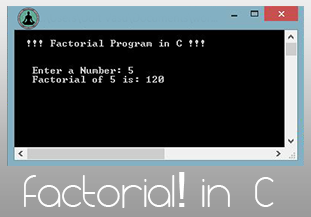# Factorial Program In C

### What is Factorial of a Number?

A mathematics term for a non-negative integer n, denoted by n!, which is the product of all positive integers less than or equal to n.
e.g- 4! = 4x3x2x1 = 24

So finding factorial of a number is a must have feature if you are willing to build a basic scientific calculator in any programming language. Here we are building factorial program in C but you can also check:

Different Methods for finding Factorial of a Number in Programming:
1. Using standard for/while loop
2. Using loop in a user defined function  (mostly used)
3. Using recursion  (best method)

#### Factorial Program in C : Using loop

This is the most common and standard method for finding factorial of a number.
Source Code:
```#include <stdio.h>
#include <conio.h>

void main(){
int num ,i,fac=1;;
printf("Enter a Number: ");
scanf("%d",&num);
for(i=num;i>0;i--){
fac = fac*i;
}
printf("Factorial of %d is: %d ",num,fac);
getch();
}```

#### Factorial Program in C : Using User Defined Function

This method is mostly used because building methods are always best practice to reduce the size and complexity of your code.
Source Code:
```#include <stdio.h>
#include <conio.h>

void main(){
int num;
printf("Enter a Number: ");
scanf("%d",&num);
printf("Factorial of %d is: %d ",num,factorial(num));
getch();
}

int factorial(int n){
int i,fac=1;
for(i=n;i>0;i--){
fac = fac*i;
}
}```

#### Factorial Program in C : Using Recursion

The most important method which every student must know to find factorial of a number using any programming language is using a recursive function (Functions are recursive if they call themselves) lets understand this method from the program given below.
```#include <stdio.h>
#include <conio.h>

void main(){
int num;
printf("Enter a Number: ");
scanf("%d",&num);
printf("Factorial of %d is: %d ",num,factorial(num));
getch();
}

int factorial(int n){
if (n<1) return 1;
else return n*factorial(n-1);
}
```

Output:So finally you learned all the three methods to build a factorial program in C.